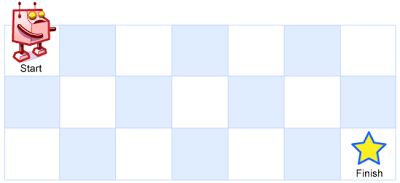# 题目1 2 3 4 5 6 7  输入: m = 3, n = 2 输出: 3 解释: 从左上角开始，总共有 3 条路径可以到达右下角。 1. 向右 -> 向右 -> 向下 2. 向右 -> 向下 -> 向右 3. 向下 -> 向右 -> 向右 

 1 2  输入: m = 7, n = 3 输出: 28 

• 1 <= m, n <= 100
• 题目数据保证答案小于等于 2 * 10 ^ 9

# 题解

  1 2 3 4 5 6 7 8 9 10 11 12 13 14 15 16 17 18 19 20  void interUniquePaths(int m, int n, int x, int y, int &count) { if (x == m - 1 && y == n - 1) { count += 1; return; } if (x < m - 1) { interUniquePaths(m, n, x + 1, y, count); } if (y < n - 1) { interUniquePaths(m, n, x, y + 1, count); } } int uniquePaths(int m, int n) { int count = 0; interUniquePaths(m, n, 0, 0, count); return count; } 

0 1 2
0 2 2 1
1 1 1 0

0 1 2
0 1 1 1
1 1 2 3

  1 2 3 4 5 6 7 8 9 10 11 12 13 14 15 16 17 18 19 20  int uniquePaths(int m, int n) { int **matrix = new int*[n]; for (int i = 0; i < n; i++) { matrix[i] = new int[m]; memset(matrix[i], 0, sizeof(int) * m); } for (int i = 0; i < n; i++) { for (int j = 0; j < m; j++) { if (i > 0 && j > 0) { matrix[i][j] = matrix[i - 1][j] + matrix[i][j - 1]; } else { matrix[i][j] = 1; } } } return matrix[n - 1][m - 1]; }